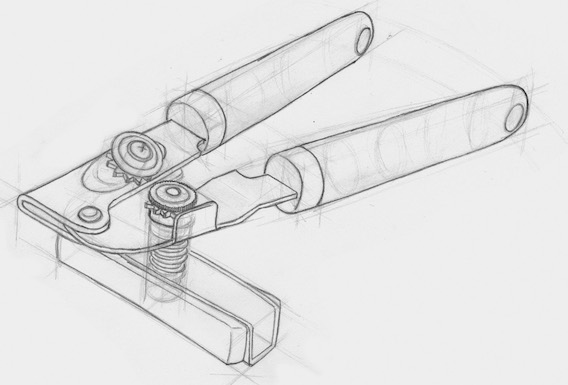# Applicative Functor

## 应用 applicative functor

``````// 这样是行不通的，因为 2 和 3 都藏在瓶子里。
//NaN

// 使用可靠的 map 函数试试
``````

``````Container.of(2).chain(function(two) {
});
``````

## 瓶中之船`ap` 就是这样一种函数，能够把一个 functor 的函数值应用到另一个 functor 的值上。把这句话快速地说上 5 遍。

``````Container.of(add(2)).ap(Container.of(3));
// Container(5)

// all together now
// Container(5)
``````

``````Container.prototype.ap = function(other_container) {
return other_container.map(this.__value);
}
``````

applicative functor 是实现了 `ap` 方法的 pointed functor

``````F.of(x).map(f) == F.of(f).ap(F.of(x))
``````

``````Maybe.of(add).ap(Maybe.of(2)).ap(Maybe.of(3));
// Maybe(5)

``````

## 协调与激励

``````// Http.get :: String -> Task Error HTML

var renderPage = curry(function(destinations, events) { /* render page */  });

// Task("<div>some page with dest and events</div>")
``````

``````// 帮助函数：
// ==============
//  \$ :: String -> IO DOM
var \$ = function(selector) {
return new IO(function(){ return document.querySelector(selector) });
}

//  getVal :: String -> IO String
var getVal = compose(map(_.prop('value')), \$);

// Example:
// ===============
//  signIn :: String -> String -> Bool -> User

// IO({id: 3, email: "gg@allin.com"})
``````

`signIn` 是一个接收 3 个参数的 curry 函数，因此我们需要调用 `ap` 3 次。在每一次的 `ap` 调用中，`signIn` 就收到一个参数然后运行，直到所有的参数都传进来，它也就执行完毕了。我们可以继续扩展这种模式，处理任意多的参数。另外，左边两个参数在使用 `getVal` 调用后自然而然地成为了一个 `IO`，但是最右边的那个却需要手动 `lift`，然后变成一个 `IO`，这是因为 `ap` 需要调用者及其参数都属于同一类型。

## lift

（译者注：此处原标题是“Bro, do you even lift?”，是一流行语，发源于健身圈，指质疑别人的健身方式和效果并显示优越感，后扩散至其他领域。再注：作者书中用了不少此类俚语或俗语，有时并非在使用俚语的本意，就像这句，完全就是为了好玩。另，关于 lift 的概念可参看第 8 章。）

``````var liftA2 = curry(function(f, functor1, functor2) {
return functor1.map(f).ap(functor2);
});

var liftA3 = curry(function(f, functor1, functor2, functor3) {
return functor1.map(f).ap(functor2).ap(functor3);
});

//liftA4, etc
``````

`liftA2` 是个奇怪的名字，听起来像是破败工厂里挑剔的货运电梯，或者伪豪华汽车公司的个性车牌。不过你要是真正理解了，那么它的含义也就不证自明了：让那些小代码块发生 lift，成为 applicative functor 中的一员。

``````// checkEmail :: User -> Either String Email
// checkName :: User -> Either String String

//  createUser :: Email -> String -> IO User
var createUser = curry(function(email, name) { /* creating... */ });

Either.of(createUser).ap(checkEmail(user)).ap(checkName(user));
// Left("invalid email")

liftA2(createUser, checkEmail(user), checkName(user));
// Left("invalid email")
``````

`createUser` 接收两个参数，因此我们使用的是 `liftA2`。上述两个语句是等价的，但是使用了 `liftA2` 的版本没有提到 `Either`，这就使得它更加通用灵活，因为不必与特定的数据类型耦合在一起。

``````liftA2(add, Maybe.of(2), Maybe.of(3));
// Maybe(5)

liftA2(renderPage, Http.get('/destinations'), Http.get('/events'))
// Task("<div>some page with dest and events</div>")

// IO({id: 3, email: "gg@allin.com"})
``````

## 操作符

``````-- haskell
add <\$> Right 2 <*> Right 3
``````
``````// JavaScript
``````

`<\$>` 就是 `map`（亦即 `fmap`），`<*>` 不过就是 `ap`。这样的语法使得开发者可以以一种更自然的风格来书写函数式应用，而且也能减少一些括号。

# 免费开瓶器``````// 从 of/ap 衍生出的 map
X.prototype.map = function(f) {
return this.constructor.of(f).ap(this);
}
``````

monad 可以说是处在食物链的顶端，因此如果已经有了一个 `chain` 函数，那么就可以免费得到 functor 和 applicative：

``````// 从 chain 衍生出的 map
X.prototype.map = function(f) {
var m = this;
return m.chain(function(a) {
return m.constructor.of(f(a));
});
}

// 从 chain/map 衍生出的 ap
X.prototype.ap = function(other) {
return this.chain(function(f) {
return other.map(f);
});
};
``````

## 定律

``````  var tOfM = compose(Task.of, Maybe.of);

liftA2(_.concat, tOfM('Rainy Days and Mondays'), tOfM(' always get me down'));
// Task(Maybe(Rainy Days and Mondays always get me down))
``````

### 同一律（identity）

``````// 同一律
A.of(id).ap(v) == v
``````

``````var v = Identity.of("Pillow Pets");
Identity.of(id).ap(v) == v
``````

`Identity.of(id)` 的“无用性”让我不禁莞尔。这里有意思的一点是，就像我们之前证明了的，`of/ap` 等价于 `map`，因此这个同一律遵循的是 functor 的同一律：`map(id) == id`

### 同态（homomorphism）

``````// 同态
A.of(f).ap(A.of(x)) == A.of(f(x))
``````

``````Either.of(_.toUpper).ap(Either.of("oreos")) == Either.of(_.toUpper("oreos"))
``````

### 互换（interchange）

``````// 互换
v.ap(A.of(x)) == A.of(function(f) { return f(x) }).ap(v)
``````

``````var v = Task.of(_.reverse);
var x = 'Sparklehorse';

``````

### 组合（composition）

``````// 组合
A.of(compose).ap(u).ap(v).ap(w) == u.ap(v.ap(w));
``````
``````var u = IO.of(_.toUpper);
var v = IO.of(_.concat("& beyond"));
var w = IO.of("blood bath ");

IO.of(_.compose).ap(u).ap(v).ap(w) == u.ap(v.ap(w))
``````

## 总结

Chapter 11: Traversable/Foldable Functors

## 练习

``````require('./support');
var _ = require('ramda');

// 模拟浏览器的 localStorage 对象
var localStorage = {};

// 练习 1
// ==========
// 写一个函数，使用 Maybe 和 ap() 实现让两个可能是 null 的数值相加。

//  ex1 :: Number -> Number -> Maybe Number
var ex1 = function(x, y) {

};

// 练习 2
// ==========
// 写一个函数，接收两个 Maybe 为参数，让它们相加。使用 liftA2 代替 ap()。

//  ex2 :: Maybe Number -> Maybe Number -> Maybe Number
var ex2 = undefined;

// 练习 3
// ==========
// 运行 getPost(n) 和 getComments(n)，两者都运行完毕后执行渲染页面的操作。（参数 n 可以是任意值）。

var makeComments = _.reduce(function(acc, c){ return acc+"<li>"+c+"</li>" }, "");
var render = _.curry(function(p, cs) { return "<div>"+p.title+"</div>"+makeComments(cs); });

//  ex3 :: Task Error HTML
var ex3 = undefined;

// 练习 4
// ==========
// 写一个 IO，从缓存中读取 player1 和 player2，然后开始游戏。

localStorage.player1 = "toby";
localStorage.player2 = "sally";

var getCache = function(x) {
return new IO(function() { return localStorage[x]; });
}
var game = _.curry(function(p1, p2) { return p1 + ' vs ' + p2; });

//  ex4 :: IO String
var ex4 = undefined;

// 帮助函数
// =====================

function getPost(i) {
return new Task(function (rej, res) {
setTimeout(function () { res({ id: i, title: 'Love them futures' }); }, 300);
});
}